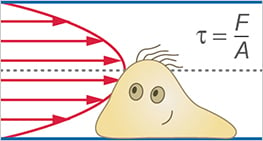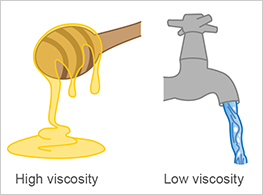# Glossary: Cells Under Flow

Shear stress is a force that acts on a surface when a solid object is pulled across the surface, or a liquid flows over it. It acts parallel to the surface, in the direction in which the object (or fluid) is moving. Shear stress (τ) is defined as force per area (τ = F/A, where τ = the shear stress, F = the force applied, and A = the cross-sectional area of the material surface).

Shear stress in fluids/flow profile: A fluid flowing through a channel can be seen as a stack of fluid layers moving on top of each other. The shear stress is caused by the friction between the layers, due to the fluid’s viscosity. The friction between the layers results in the velocity distribution, the flow profile. The viscosity determines how high the friction between the layers is.Wall shear stress (WSS) is present directly at the boundary layer from the channel surface to the first liquid layer when liquid flows through a channel. This is the force experienced by the cells and which influences their behavior, morphology, and physiology. WSS is directly related to the viscosity of the fluid and the shear rate.

Shear rate is defined as the change in velocity, at which one fluid moves over an adjacent layer. The shear rate is determined by both the vessel cross-section and the flow rate, measured in reciprocal seconds (s-1). The shear rate is an important parameter in rolling adhesion experiments, as it indicates how fast the cells roll over the surface; or how long the contact time is to the adherent cells.

Viscosity (strictly speaking, dynamic viscosity) is the property of liquids that describes their fluidity. It decisively determines how easily liquid layers can slide over each other and is an important parameter for calculating shear stress. Water, for example, has a lower viscosity than honey. In Newtonian fluids (e.g., water, cell culture medium), the viscosity is independent of the flow rate.Flow rate is the volume flow through a channel in a given time. The flow rate alone does not indicate the force applied to the adherent cells, but it is needed for the shear stress calculation. The flow rate (Φ) is defined as a volume per time (e.g., ml/min).

The Reynolds number (Re) describes whether a fluid flow is laminar or turbulent. The Reynolds number is given by the ratio of inertial forces to friction forces in in a fluid. This value is dimensionless. Laminar flow occurs at low Reynolds numbers whereas turbulent flow occurs at high Reynolds numbers. The critical Reynolds number, which indicates laminar flow in pipes and biological vessels, is Re=2000. A Reynolds number of above approximately Re 4000 is most likely to represent a turbulent flow.

Please read the section “The Different Types of Flow” for a description of unidirectional, pulsatile, and oscillatory laminar flow, as well as turbulent flow.